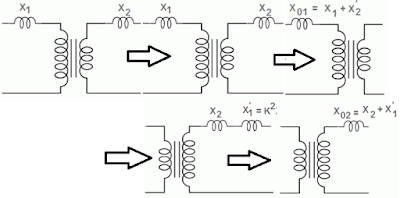WAZIPOINT Engineering Science & Technology: Transformer Equivalent Resistance

## Thursday, June 2, 2022

### Transformer Equivalent ResistanceFig-1: Transformer Equivalent ResistanceFig-2: Transformer Equivalent Reactance

## How to Calculate the Transformer Equivalent Resistance and Reactance?

The transformer’s impedance is given in percentage or per unit system. It is because of the reason that the impedance value for the transformer is represented as two values one wrt to low voltage winding and the other is high voltage winding. Both values are different but represent the very same physical quantity.

Transformer equivalent impedance contains two-part, one is equivalent resistance and the other is equivalent reactance. The vector sum of these two parts is known as equivalent impedance.

### Transformer Equivalent Resistance

Considering the figure-1 the above
Let
R1 = primary winding resistance;
R2 = secondary winding resistance;
K = transformation ratio.

The equivalent resistance of the transformer referred to as the primary is represented by R01.

Therefore, R01 = R1 + R2' = R1 + R2/K2.

The equivalent resistance of the transformer referred to as the secondary is represented by R02.

Therefore, R02 = R2 + R1' = R2 + K2R1.

### Transformer Equivalent Reactance

Considering the figure-2 the above
Let
X1 = primary winding reactance;
X2= secondary winding reactance;
K = transformation ratio.

The equivalent reactance of the transformer referred to as the primary is represented by X01.

Therefore, X01 = X1 + X2' = X1 + X2/K2

The equivalent reactance of the transformer referred to as the secondary is represented by X02.

Therefore, X02 = X2 + X1' = X2 + K2X1.

#### The impedance of the Transformer

Impedance(Z) = Resistance(R) + j Reactance(X)
The primary impedance Z1 = R1 + jX1
And secondary impedance Z2 = R2 + jX2

The transfer of impedances takes place on the same lines as that of the resistances. The transfer of impedances can take place from primary to secondary and vice versa.

Percentage Resistance is the resistance drop in volts at rated current and frequency as a percentage of the rated voltage.
%R = ( IR/V)*100

Percentage Reactance is the reactance drop in volts at rated current and frequency as a percentage of the rated voltage.
%X = ( IX/V)*100

Percentage impedance is nothing but a measure of the volt drops when the transformer is on full load due to the winding resistance and leakage reactance expressed as a percentage of the rated voltage.
%Z = ( IZ/V)*100

Z01 = (R012 + X012)1/2 impedance referred to primary side.
Z02 = (R022 + X022)1/2  impedance referred to secondary side.

The important advantages of expressing resistance and reactance of a transformer in percentage are that the percentage resistance and percentage reactance have the same values whether determined and referred to as primary or secondary whereas expressed in ohms they have different values when referred to as primary and secondary.